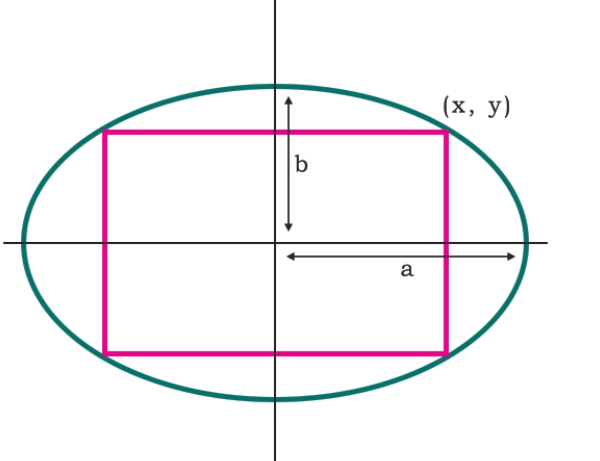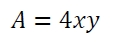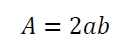# Area of Largest rectangle that can be inscribed in an Ellipse?

Here we will see the area of largest rectangle that can be inscribed in an ellipse. The rectangle in ellipse will be like below −The a and b are the half of major and minor axis of the ellipse. The upper right corner of the rectangle is (x, y). So the area isNow, after making this equation as f(x) and maximizing the area, we will get the area as## Example

#include <iostream>
#include <cmath>
using namespace std;
float area(float a, float b) {
if (a < 0 || b < 0 ) //if the valuse are negative it is invalid
return -1;
float area = 2*a*b;
return area;
}
int main() {
float a = 10, b = 8;
cout << "Area : " << area(a, b);
}

## Output

Area : 160